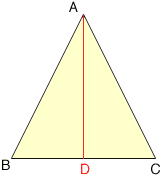Quandaries and Queries Could you please tell me the formular for me to calculate the height of a triangle. I have the angles and side lengths. I am trying to calculate the height of an isosceles triangle, does this make a difference from a normal triangle or is the formular the same. Thank you Dean age 14 Hi Dean, The fact that the triangle is iscosceles does make a difference. In the diagram below the line segment AD is perpendicular to BC and, since |AB| = |AC|, we know that D is the midpoint of BC. Thus you know the length of BD.Now you can use the theorem of Pythagoras with the triangle ABD to find the length of AD, which is the height of the triangle. That is |AB|2 = |BD|2 + |DA|2 and you know |AB| and |BD| so you can find |AD|. If |AB| is not equal to |AC| then D is not the midpoint of BC so this method doesn't work. In this case you can use trigonometry. The sine of the angle at B is  |AD|/|AB| and thus |AD| = |AB| sin(B) Penny Go to Math Central